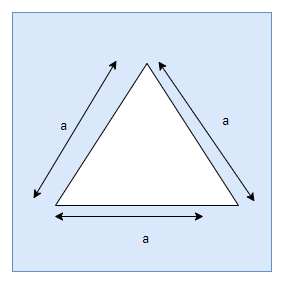# Area of Equilateral Triangle Calculator

## Area of Triangle Calculator(Heron Formula)

Note
• Enter the values of Side of the triangle or Area of the Triangle
• Click on the calculate button to get the value of empty

Formula And concept used
if a is side of the triangle,then Area,Height and Perimeter is given
$A = \frac {a^2 \sqrt 3}{4}$
$p =3a$
$h = \frac { a \sqrt 3}{2}$

## What is Equilateral TriangleEquilateral triangle is a triangle where all the side are equal. The perpendicular from the vertices to the Opposite sides divides the side.
Area of the Equilateral triangle can be calculated using Heron formula which is
$A = \sqrt { s(s-a)(s-b)(s-c)}$ where $s = \frac {a +b +c}{2}$
Now for equilateral triangle
a=b=c=a
Therefore
$s = \frac {a +b +c}{2}= \frac {3a}{2}$
$A=\sqrt { s(s-a)(s-b)(s-c)} = \sqrt {\frac {3a}{2} ( \frac {3a}{2} -a) ( \frac {3a}{2} -a)( \frac {3a}{2} -a)} = \frac {a^2 \sqrt 3}{4}$
Perimeter of the Equilateral triangle is given by
$p = 3a$
Now Height can be calculated
We know that Area is also given as
$A = \frac {1}{2} ah$
Therefore
$\frac {1}{2} ah= \frac {a^2 \sqrt 3}{4}$
$h = \frac { a \sqrt 3}{2}$

Example of Few questions where you can use this Area of Equilateral Triangle Calculator
Question 1
Find the Area ,Height and Perimeter of a Equilateral Triangle whose side is.
i. 4 cm
ii. 12 cm
Solution
(i) a = 4 cm
Area of Equilateral Triangle is calculated as
$A= \frac {a^2 \sqrt 3}{4} = \frac {4^2 \sqrt 3}{4}= 4 \sqrt {3} \ cm$
Height is calculated as
$h = \frac { a \sqrt 3}{2}= \frac { 4 \sqrt 3}{2}=2 \sqrt {3} \ cm$
Perimeter of the Equilateral triangle is given by
$p = 3a = 3 \times 4 = 12 \ cm$
(ii) a = 12 cm
Area of Equilateral Triangle is calculated as
$A= \frac {a^2 \sqrt 3}{4} = \frac {(12)^2 \sqrt 3}{4}= 36 \sqrt {3} \ cm$
Height is calculated as
$h = \frac { a \sqrt 3}{2}= \frac { 12 \sqrt 3}{2}=6 \sqrt {3} \ cm$
Perimeter of the Equilateral triangle is given by
$p = 3a = 3 \times 12 = 36 \ cm$

Question 2
Find the Side,Perimeter and Height of a Equilateral Triangle whose Area is.
i. $400 \sqrt 3$ cm2
ii. $100 \sqrt 3$ cm2
Solution
(i) A = $300 \sqrt 3$ cm2
Area of Equilateral Triangle is calculated as
$A= \frac {a^2 \sqrt 3}{4} = \frac {4^2 \sqrt 3}{4}= 4 \sqrt {3} \ cm$
Rearranging for Side
$a = \sqrt {\frac {4A}{\sqrt 3}}= \sqrt { \frac {4 \times 400 \sqrt 3}{\sqrt 3}}= 40 \ cm$
Height is calculated as
$h = \frac { a \sqrt 3}{2}= \frac { 40 \sqrt 3}{2}=20 \sqrt {3} \ cm$
Perimeter of the Equilateral triangle is given by
$p = 3a = 3 \times 40 = 120 \ cm$
(ii) A = $100 \sqrt 3$ cm2
Area of Equilateral Triangle is calculated as
$A= \frac {a^2 \sqrt 3}{4} = \frac {4^2 \sqrt 3}{4}= 4 \sqrt {3} \ cm$
Rearranging for Side
$a = \sqrt {\frac {4A}{\sqrt 3}}= \sqrt { \frac {4 \times 100 \sqrt 3}{\sqrt 3}}= 20 \ cm$
Height is calculated as
$h = \frac { a \sqrt 3}{2}= \frac { 20 \sqrt 3}{2}=10 \sqrt {3} \ cm$
Perimeter of the Equilateral triangle is given by
$p = 3a = 3 \times 20 = 60 \ cm$

## How Calculator works

Side Given
• Area of Equilateral Triangle is calculated as
$A= \frac {a^2 \sqrt 3}{4}$
• Height is calculated as
$h = \frac { a \sqrt 3}{2}$
• Perimeter of the Equilateral triangle is given by
$p = 3a$

Area Given
• Side of Equilateral Triangle is calculated as
$a= \sqrt {\frac {4A}{\sqrt 3}}$
• Height is calculated as
$h = \frac { a \sqrt 3}{2}$
• Perimeter of the Equilateral triangle is given by
$p = 3a$

Perimeter Given
• Side of Equilateral Triangle is calculated as
$a= \frac {p}{3}$
• Area of Equilateral Triangle is calculated as
$A= \frac {a^2 \sqrt 3}{4}$
• Height is calculated as
$h = \frac { a \sqrt 3}{2}$

Height Given
• Side of Equilateral Triangle is calculated as
$a= \frac {2h}{\sqrt 3}$
• Area of Equilateral Triangle is calculated as
$A= \frac {a^2 \sqrt 3}{4}$
• Perimeter of the Equilateral triangle is given by
$p = 3a$